Full Text:<3773>

CLC number: TV3

On-line Access: 2013-04-03

Revision Accepted: 2013-01-09

Crosschecked: 2013-03-06

Cited: 2

Clicked: 3847

Citations:  Bibtex RefMan EndNote GB/T7714

 Journal of Zhejiang University SCIENCE A 2013 Vol.14 No.4 P.256-267 http://doi.org/10.1631/jzus.A1200196

Coupled elasto-plasticity damage constitutive models for concrete*

 Author(s):  Qiang Xu1, Jian-yun Chen1,2, Jing Li2, Gang Xu3 Affiliation(s):  1. State Key Laboratory of Coastal and Offshore Engineering, Dalian University of Technology, Dalian 116023, China; more Corresponding email(s):   xuqiang528826@163.com Key Words:  Concrete, Damage constitutive models, Hsieh-Ting-Chen four-parameter yield function, Uniaxial tension, Uniaxial compression, Gravity dam, Arch dam Share this article to： More <<< Previous Article|Next Article >>>

Qiang Xu, Jian-yun Chen, Jing Li, Gang Xu. Coupled elasto-plasticity damage constitutive models for concrete[J]. Journal of Zhejiang University Science A, 2013, 14(4): 256-267.

@article{title="Coupled elasto-plasticity damage constitutive models for concrete",
author="Qiang Xu, Jian-yun Chen, Jing Li, Gang Xu",
journal="Journal of Zhejiang University Science A",
volume="14",
number="4",
pages="256-267",
year="2013",
publisher="Zhejiang University Press & Springer",
doi="10.1631/jzus.A1200196"
}

%0 Journal Article
%T Coupled elasto-plasticity damage constitutive models for concrete
%A Qiang Xu
%A Jian-yun Chen
%A Jing Li
%A Gang Xu
%J Journal of Zhejiang University SCIENCE A
%V 14
%N 4
%P 256-267
%@ 1673-565X
%D 2013
%I Zhejiang University Press & Springer
%DOI 10.1631/jzus.A1200196

TY - JOUR
T1 - Coupled elasto-plasticity damage constitutive models for concrete
A1 - Qiang Xu
A1 - Jian-yun Chen
A1 - Jing Li
A1 - Gang Xu
J0 - Journal of Zhejiang University Science A
VL - 14
IS - 4
SP - 256
EP - 267
%@ 1673-565X
Y1 - 2013
PB - Zhejiang University Press & Springer
ER -
DOI - 10.1631/jzus.A1200196

Abstract:
The paper is to design and construct a coupled elasto-plasticity damage constitutive model for concrete. Based on the energy dissipation principle, the hsieh-Ting-Chen four-parameter yield function is used. The model can reflect different strength characteristics of concrete in tension and compression, and reduce the limitation and lacuna of the traditional damage constitutive models for concrete. Furthermore, numerical test for concrete stress-strain relation under uniaxial tension and compression is given. Moreover, the damage process of concrete gravity dam is calculated and analyzed in seismic load. Compared with other damage constitutive models, the proposed model contains only one unknown parameter and the other parameters can be found in the hsieh-Ting-Chen four-parameter yield function. The same damage evolution law, which is used for tension and compression, is good for determining stress-strain constitutive and damage characteristics in complex stress state. This coupled damage constitutive models can be applied in analyzing damage of concrete gravity dam and arch dam.

## 1.  Introduction

The theory and models of elasto-plasticity damage constitutive for concrete have been developed significantly during the last thirty years and have been documented in an increasing number of publications. These improvements in damage constitutive models for concrete and the attainment of more accurate quantification of the damage have stimulated interest in structure damage analysis. Although from a theoretical point of view the field has reached a stage where the developed methodologies are becoming widespread, the quantitative assessment and classification of damage of structure is still a complex and difficult task.

Many studies added damage factor in constitutive models to simulate the constitutive relation of concrete. The classical damage models contain the models proposed by Loland (), Krajcinovic (), Mazars and Pijaudier-Cabot (), Aifantis (), and so on. Based on this research, a rigorous series of tests has to be carried out. Ortiz () proposed a general theory for the inelasticity of concrete, which was a new, rate-independent model of distributed damage for mortar and the application of mixture theories to account for the composite nature of concrete. A numerical scheme based on nonlinear crack band theory was conducted by Wang et al. () to study the 2D seismic fracture behavior of concrete gravity dams. Dragon et al. () synthesized a continuum damage model attempting to capture salient features of progressive anisotropic degradation and accounting for frictional sliding over internal crack surfaces. Brencich and Gambarotta () formulated incremental constitutive equations for brittle materials on the grounds of a frictional microcracked elastic model. Comi and Perego () presented an isotropic damage model for concrete. The main features of the model were: limited number of constitutive parameters required; independent modelling of tension and compression behaviour by means of two damage variables and two separate activation criteria (bi-dissipative model); independent definition of tension and compression fracture energies by means of constitutive parameters which do not affect the pre-peak behaviour; consistent modelling of the unilateral effect upon transition from tension to compression. The study on a nonlinear joint element model with a coupled shear-tensile behavior for realistic finite element analysis of a dam-reservoir system was made by Ahmadi et al. (). Kuna-Ciskal and Skrzypek () analysed anisotropic damage evolution and crack propagation in the elastic-brittle materials by the concepts of continuum damage mechanics (CDM) and finite element method (FEM). Marfia et al. () studied the cyclic behavior of reinforced concrete beams taking into account the compression and tensile softening in the concrete material. A new structural damage identification method was proposed by Chen et al. () using limited test static displacement based on the grey system theory. Mirzabozorg and Ghaemian () proposed a smeared crack approach to model the static and dynamic behavior of mass concrete in 3D space. The strain-rate effects were discussed by Lin and Hu () for the earthquake safety assessment of concrete dams. Calayir and Karaton () investigated the earthquake damage response of the concrete gravity dams considering the effects of dam-reservoir interaction and selected a continuum damage model which is a second-order tensor and includes the strain softening behavior for the concrete material. Contrafatto and Cuomo () were concerned with the description and the validation of a constitutive model for concrete characterized by a combined plastic-hardening-damage-fracture dissipative criterion developed within the framework of the simple material model, so that its numerical implementation was easy and robust. Pan et al. () presented a nonlinear model for analysis of damage-cracking behavior in arch dams during strong earthquakes using a massless foundation model and viscous-spring boundary input model. Bazant et al. () conducted an improvement of the microplane model for concrete—a constitutive model in which the nonlinear triaxial behavior was characterized by relations between the stress and strain components on a microplane of any orientation under the constraint that the strains on the microplane are the projections of the macroscopic strain tensor.

These models, however, contain many parameters (Kratzig and Polling, ; Tao and Phillips, ; Ding and Yu, ), and the expressions of these damage models are complex (Peng et al., ). This paper proposes a new elasto-plasticity damage constitutive model for concrete in order to reduce the limitation and lacuna of the traditional damage constitutive models for concrete. This damage constitutive model is based on the energy dissipation principle using the Hsieh-Ting-Chen four-parameter yield function that can reflect different strength characteristics of concrete in tension and compression. Moreover, this paper gives numerical test for concrete stress-strain relation under uniaxial tension and compression. Compared with other damage constitutive models, the proposed model contains only one unknown parameter and the other parameters can be found in the Hsieh-Ting-Chen four-parameter yield function. The same damage evolution law is used to express the damage of tension and compression. It is good for determining stress-strain constitutive and damage characteristic in a complex stress state. This coupled damage constitutive model can be applied in concrete gravity dam and has high precision to describe damage of concrete gravity dam.

## 2.  Establishment of elasto-plastic damage constitutive

We attempt to establish a model which can reflect different damage characteristics between tension and compression although using the same damage function and the same parameters. The process of establishment of the elasto-plastic damage constitutive is deduced as follows.

### 2.1.  Calculation model of plastic strain

In this study, a four-parameter yield function proposed by Hsieh et al. (1983) is adopted to indicate the characteristics of tension and compression of concrete. It is given by , where a, b, c, and d are all material parameters. These parameters control the shape of the meridian stress plane and deviatoric stress plane. When a=c=0, this four-parameter yield function changes into the Drucker-Prager model. When a=c=d=0, this four-parameter yield function changes into the von Mises model. When a=b=d=0 and c=fc/f t, this four-parameter yield function changes into the Rankine model, where f c′ and f t are the compressive and tensile strengths, respectively. I 1 is the first invariant of stress tensor, J 2 is the second invariant of deviator stress tensor, σ 1 is the first principal stress, and κ is the strengthen parameter.

As simple as possible, the damage threshold function is equivalent to the yield function, and the criteria of loading and unloading are given by where , where σij and f are the stress tensor and yield function, respectively.

Then the calculation of plastic strain is the same as the classical plastic theory. In this section, it is briefly introduced. Plastic potential function F is adopted as the form of yield function f, which is written as . For , and , where , then substituting Eqs. (3), (6), and (7) into Eq. (5), we have , and then the plastic strain can be calculated. Here, σkl is also the stress tensor, and the subscriptions k and l appear twice to indicate summation formula for two expressions.

### 2.2.  Calculation model of damage factor

Based on the dissipation inequality of Clausius-Duhem, we have , where ψ is the Helmholtz free energy of unit volume.

Because it has the direct relation between ψ and elastic strain tensor (, in which is the plastic strain tensor), it is assumed that ψ can be decomposed into elastic and plastic Helmholtz free energy of unit volume (ψ e and ψ p). Then, , where D + and D are the damage factors of tension and compression, respectively. r + and r are the damage weight values of tension and compression, respectively.

r + and r are given by where is the equivalent stress, .

The damage factor D is defined as , where is the effective area for actual load, and A is the nominal area (initial area).

The relation between normal stress σ and effective stress can be expressed as , where σ and are the stresses on nominal area A and effective area , respectively.

Then we can obtain: .

It is assumed that the strain of damaged material under the normal stress σ and the strain of non-damaged material under the effective stress are equivalent. The relation can be expressed as , where E and are the elastic modulus of non-damaged and damaged materials, respectively.

Then we have , and .

Based on the consistency condition of yield function, we have , then , , where C 0 klij and Cklij are the non-damaged and damaged material stiffness tensors, respectively.

Substituting Eqs. (20) and (21) into Eq. (18), Eq. (9) can be rewritten as , then .

Because Eq. (23) is always established aiming at arbitrary , then . ψ e is regarded as strain energy, then we have .

Thus, .

For , then substituting Eq. (27) into Eq. (26), we have .

Then ψ p can be obtained from Eq. (28) (ignoring the other unrelated items with κ) .

It is defined as , where Y ± is the strain energy release rate of the damage factor of tension or compression.

For , then .

As simple as possible, the dissipation potential ψ * is expressed as , where w is the undetermined coefficient.

Substituting Eqs. (30) and (33) into Eq. (34), we can obtain: .

Substituting four-parameter yield function f(I 1,J 2,σ 1,κ) into Eq. (34), we have , where is the elastic strain, is the first principal elastic strain, and is the equivalent elastic strain. D ± can be calculated as an incremental form according to Eq. (35).

### 2.3.  Calculation model of elasto-plastic damage constitutive equation

From the above analysis, the elasto-plastic damage constitutive equation can be written as ,

Then the elasto-plastic damage constitutive model can be established, and it can calculate the damage factor of tension or compression that are coupled and plastic strain.

## 3.  Numerical simulation

### 3.1.  Model applied in concrete

This section presents a numerical simulation for concrete. The compressive strength fc=12.8 MPa, elastic modulus E=26.7 GPa, Poisson’s ratio λ=0.25. The parameters a, b, c, and d are 2.0006, 0.9814, 9.1312, and 0.22120, respectively, and w=1×10−6. The complex stress path of unload and then load is adopted in the process of uniaxial tension and compression.

A comparison of the Mazars model (Mazars and Pijaudier-Cabot, ), standard GB50010-2002, experimental data from (Zhang, ) and the model proposed in this study is shown in Fig. 1. From Fig. 1, it can be observed that the proposed constitutive models can reflect different strength characteristics of concrete in tension and compression although using the same expressions and parameters. The stress-strain relation of the proposed model is similar to the Mazars model, GB50010-2002 and experiment data. It shows that the proposed model can not only describe the stress-strain relation of concrete well under uniaxial tension and compression, but also the strength softening, plastic deformation and elastic modulus decrease of concrete.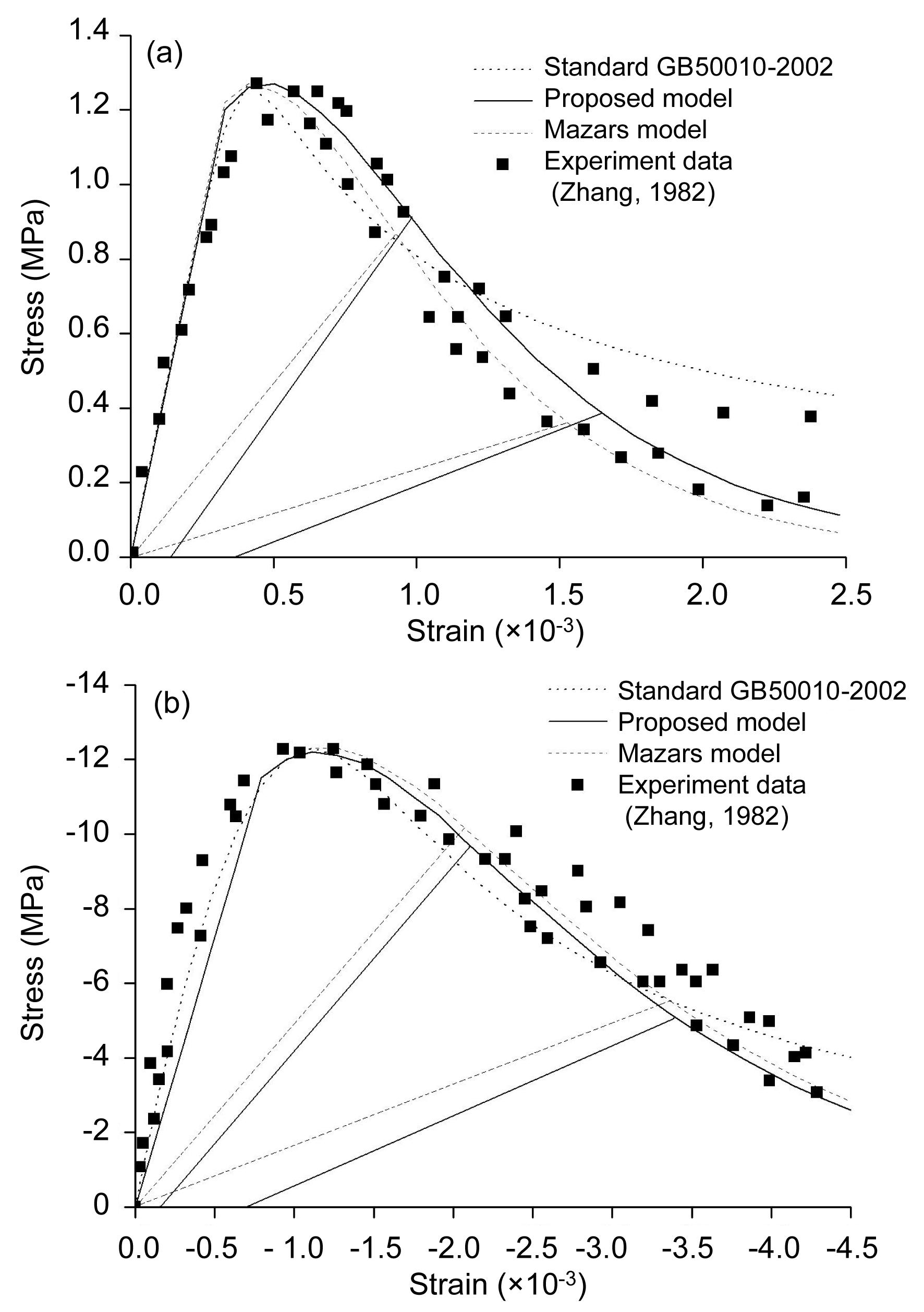Fig.1
Stress-strain curves of uniaxial tension (a) and compression (b) (unit: MPa)

The damage model in software Abaqus/CAE in complex stress state is proven and still effective. To verify the reliability of the damage model in complex stress state, which is proposed in this paper, a comparison of the computed results by the damage model in software Abaqus/CAE and by proposed damage model is made. Thus, some numerical experiments on concrete systems, which are concrete gravity dam and arch dam according to subsections 3.2 and 3.3, subjected to multiaxial stress state are made in advance to verify the applicability of the proposed model.

### 3.2.  Model applied in concrete gravity dam

The gravity dam is 160 m high, the normal pool level (NPL) is 155 m deep, and the level of the back of the dam is 10 m deep. The elevations of upstream and downstream broken-line sloping surface relative to foundation plane are 80 m and 140 m, respectively. The density of the dam ρ=2450 kg/m3, and Poisson’s ratio v=0.18. The elastic modulus of concrete E= 25 GPa. The compressive strength fc=12.8 MPa. The parameters a, b, c, and d are 2.0006, 0.9814, 9.1312, and 0.22120, respectively, and w=1×10−6. The damping ratio of dam ζ=0.05. The density of rock foundation ρ=2700 kg/m3, and Poisson’s ratio v=0.25. The elastic modulus of rock foundation E=20 GPa. Applied loads include gravity, hydrostatic, uplift pressure and seismic acceleration whose horizontal peak acceleration is 0.25g. The normalized acceleration time course is shown in Fig. 2.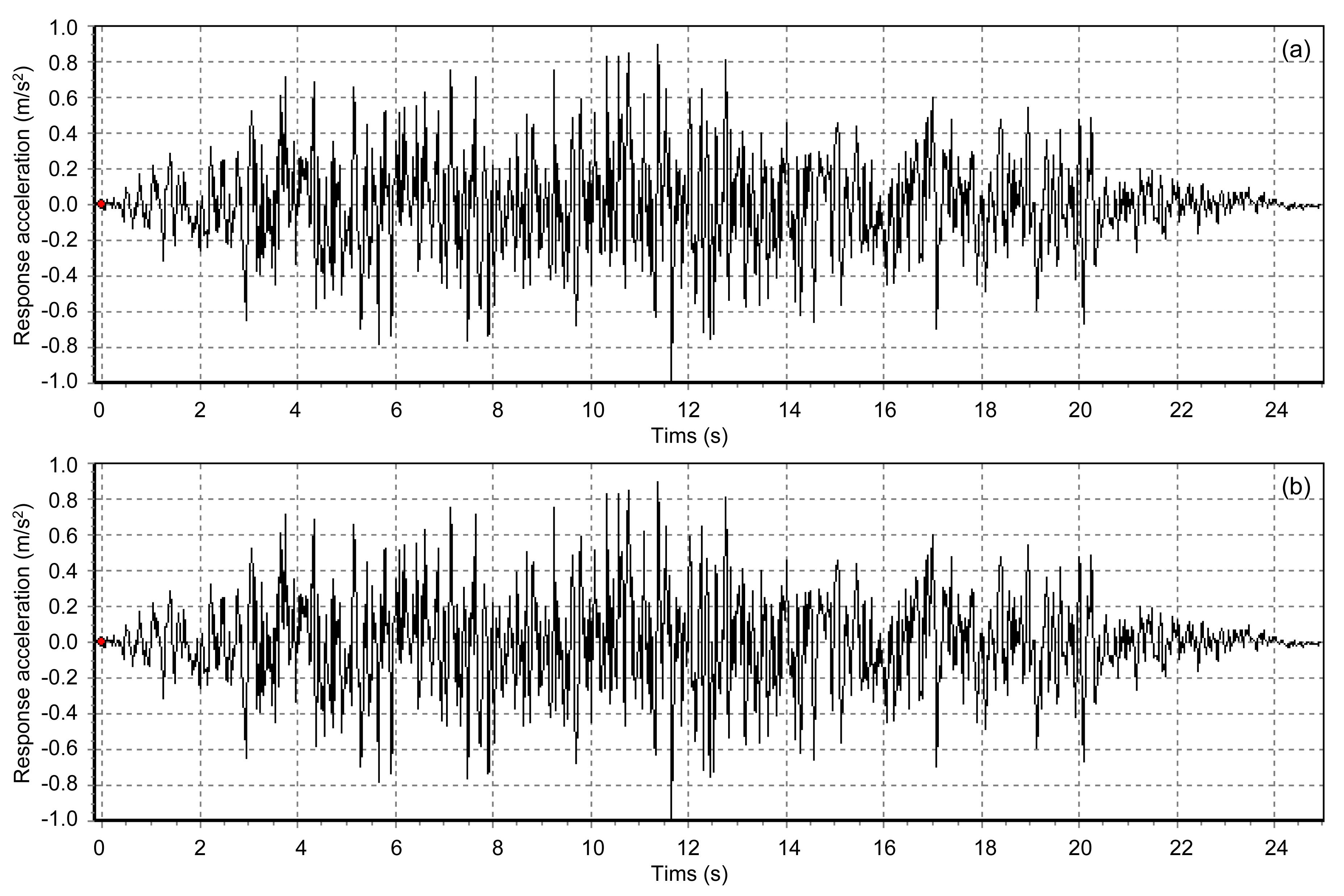Fig.2
Normalized acceleration time course of acceleration along the river (a) and in vertical direction (b) (unit: m/s2)

The dam system and monitoring points are as shown in Fig. 3. The maximum first principle stress distribution of the dam whose material is regarded as linear elastic constitutive relations is given in Fig. 4. It can be observed that the maximum principal tensile stress is concentrated in the upstream and downstream slopes and the heel of the concrete gravity dam. The damage factor time course of monitoring points can be found in Fig. 5. We can find that the development of the damage factor is concentrated in the stationary phase of the seismic load. Fig. 6 shows the damage factor contour maps of the dam at t=5.00 s, 10.00 s and 15.00 s, where the region of damage is similar to the maximum principal tensile stress. The comparison of horizontal and vertical displacements of the dam head in elastic-plastic damage and linear elastic constitutive relation is shown in Figs. 7a and 7b, respectively. It is clear that the irreversible deformation in the horizontal direction is lower than the vertical direction. Compared Fig. 6 with Fig. 8, we can find that the distributions of damage calculated by the damage model proposed in software Abaqus/CAE and in this paper are similar. It is assumed that the value of damage calculated by the damage model in software Abaqus/CAE is the true value. The value of damage calculated by the damage model proposed in this paper is smaller than that in software Abaqus/CAE, which is smaller than the true value.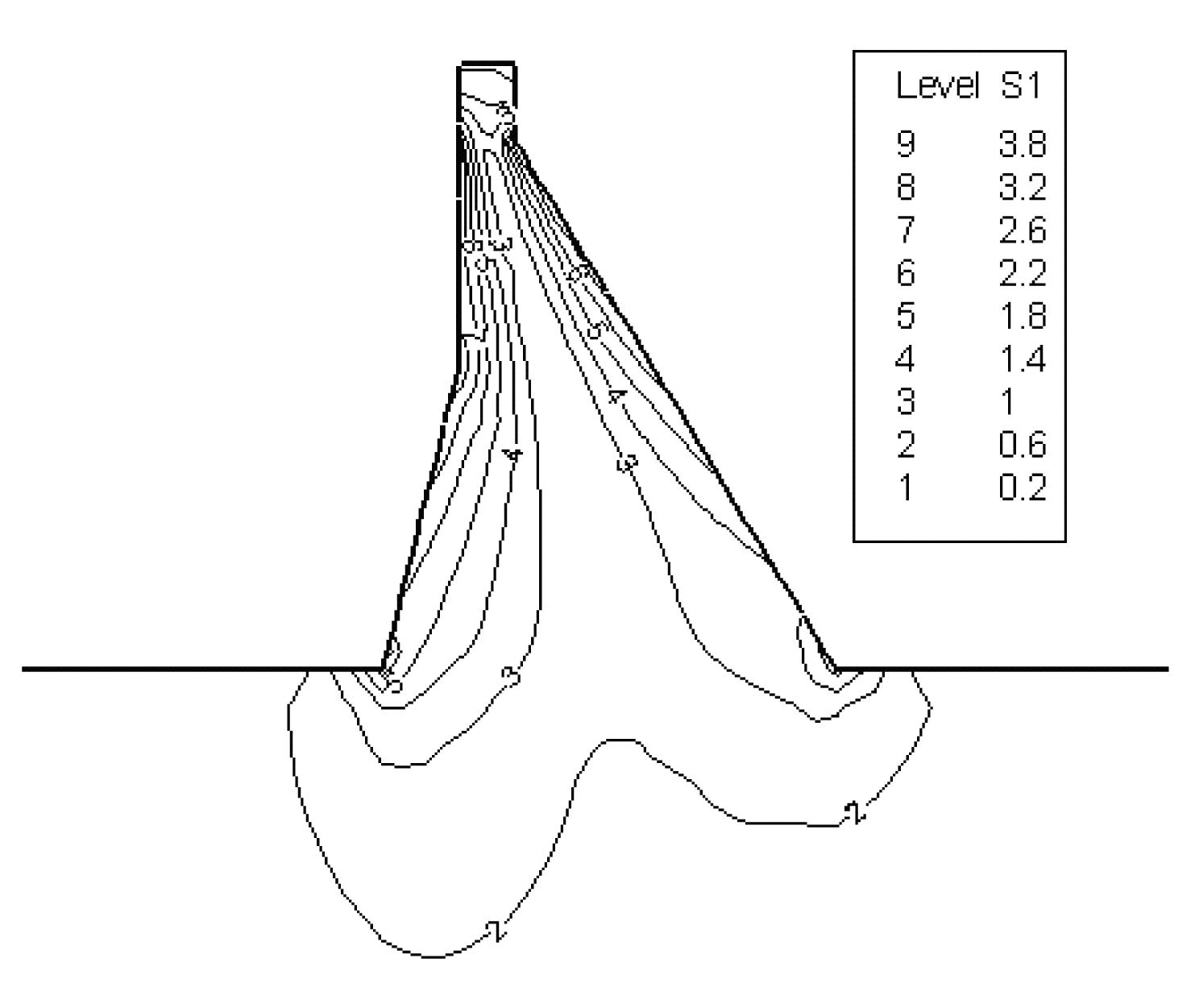Fig.4
Maximum first principle stress distribution of the slice of dam whose material is regarded as linear elastic constitutive relations (unit: MPa)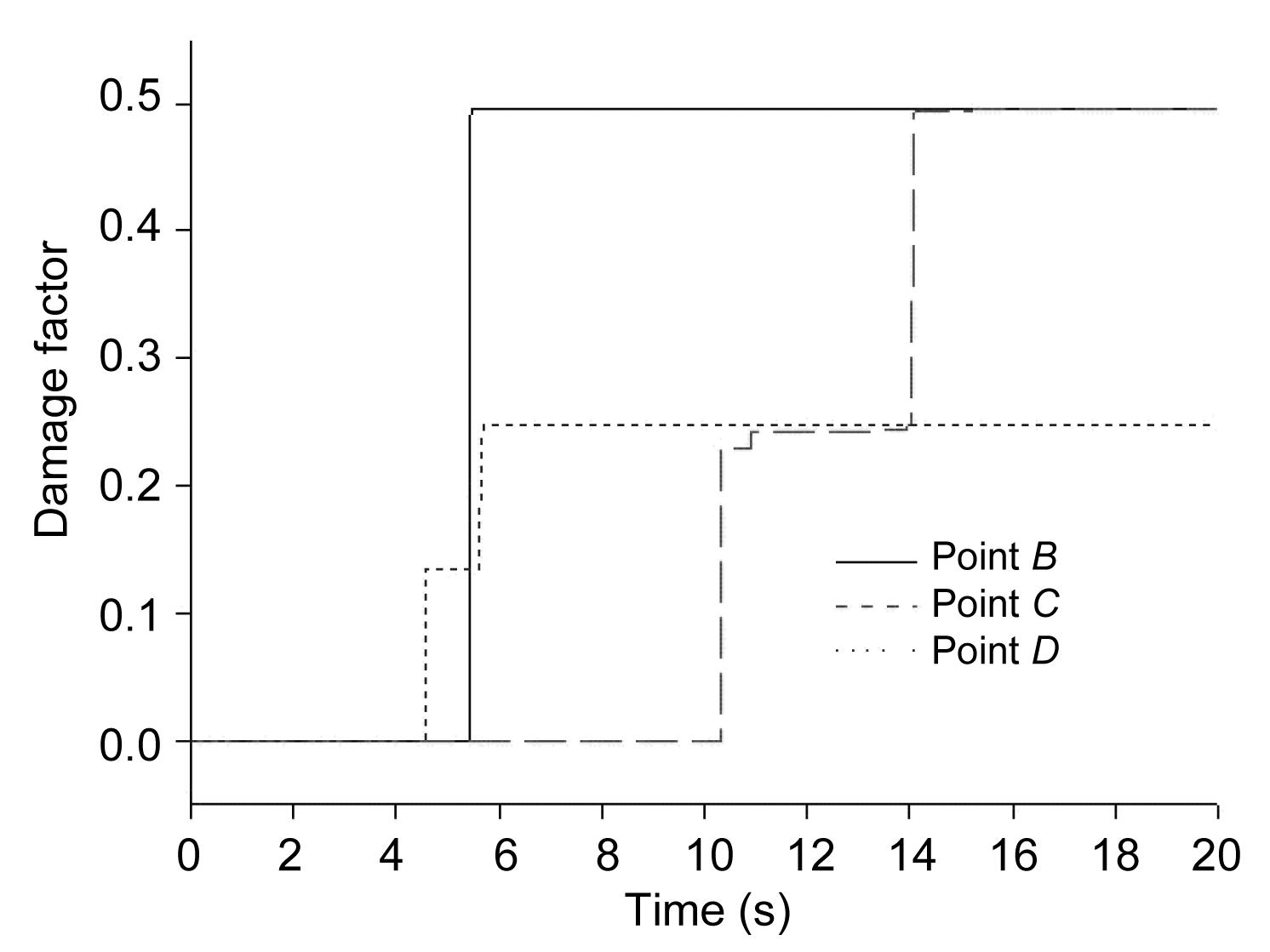Fig.5
Damage factor time course of monitoring points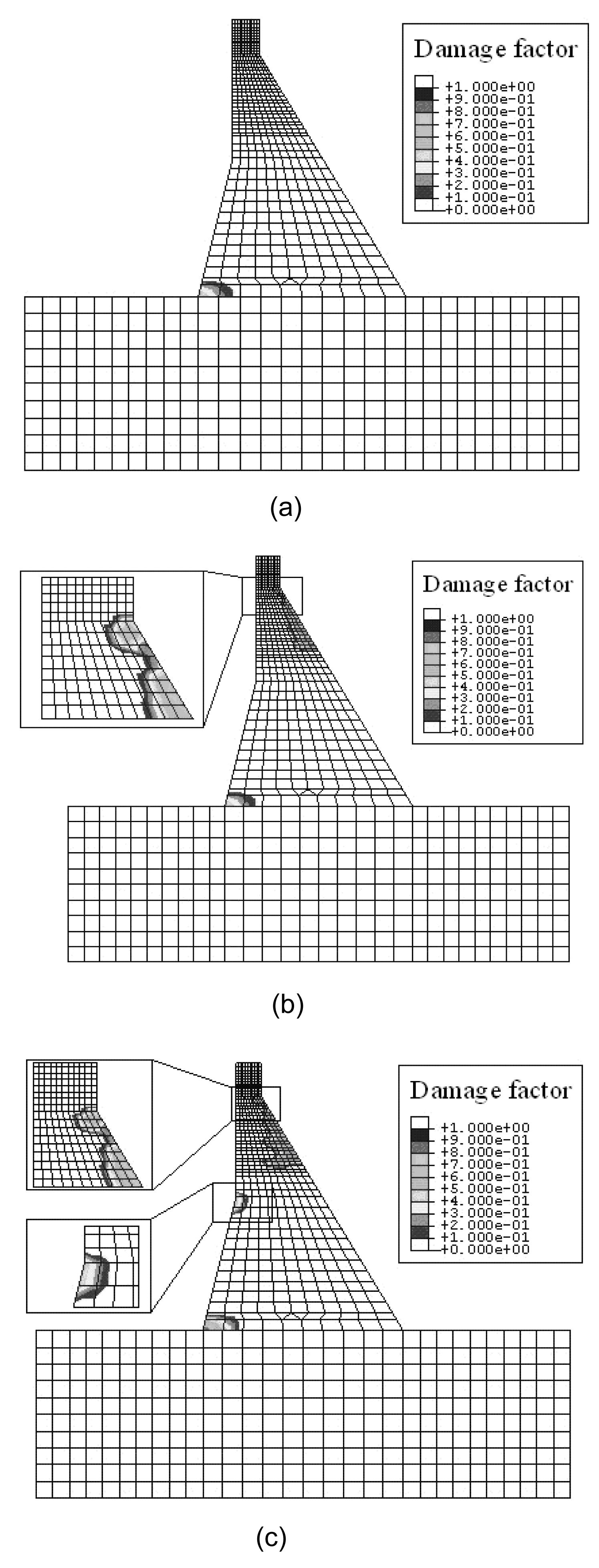Fig.6
Damage factor contour map of the slice of dam at t=5.00 s (a), 10.00 s (b) and 15.00 s (c)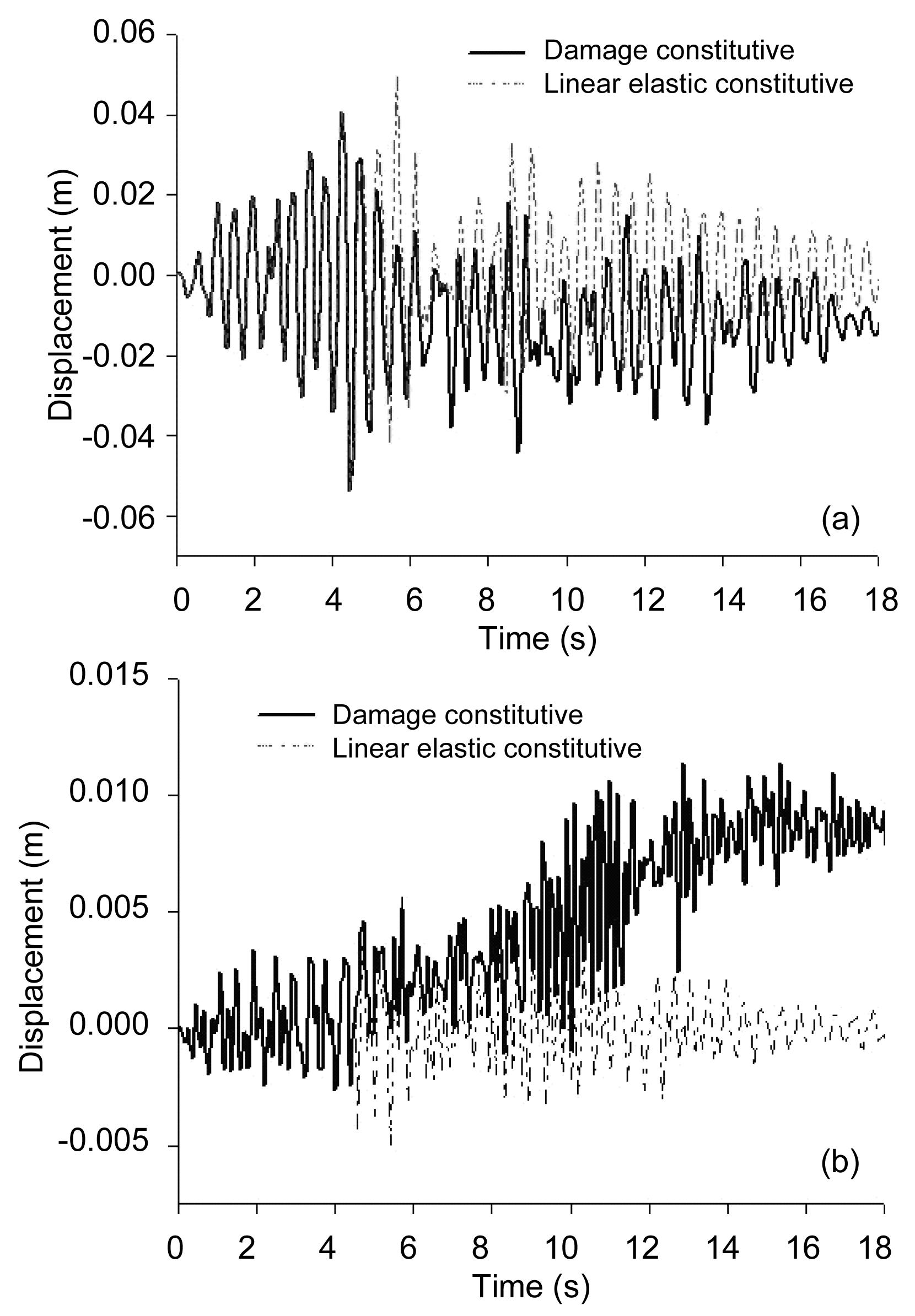Fig.7
Comparison of horizontal displacement (a) and vertical displacement (b) of the slice of dam head in elastic-plastic damage and linear elastic constitutive relation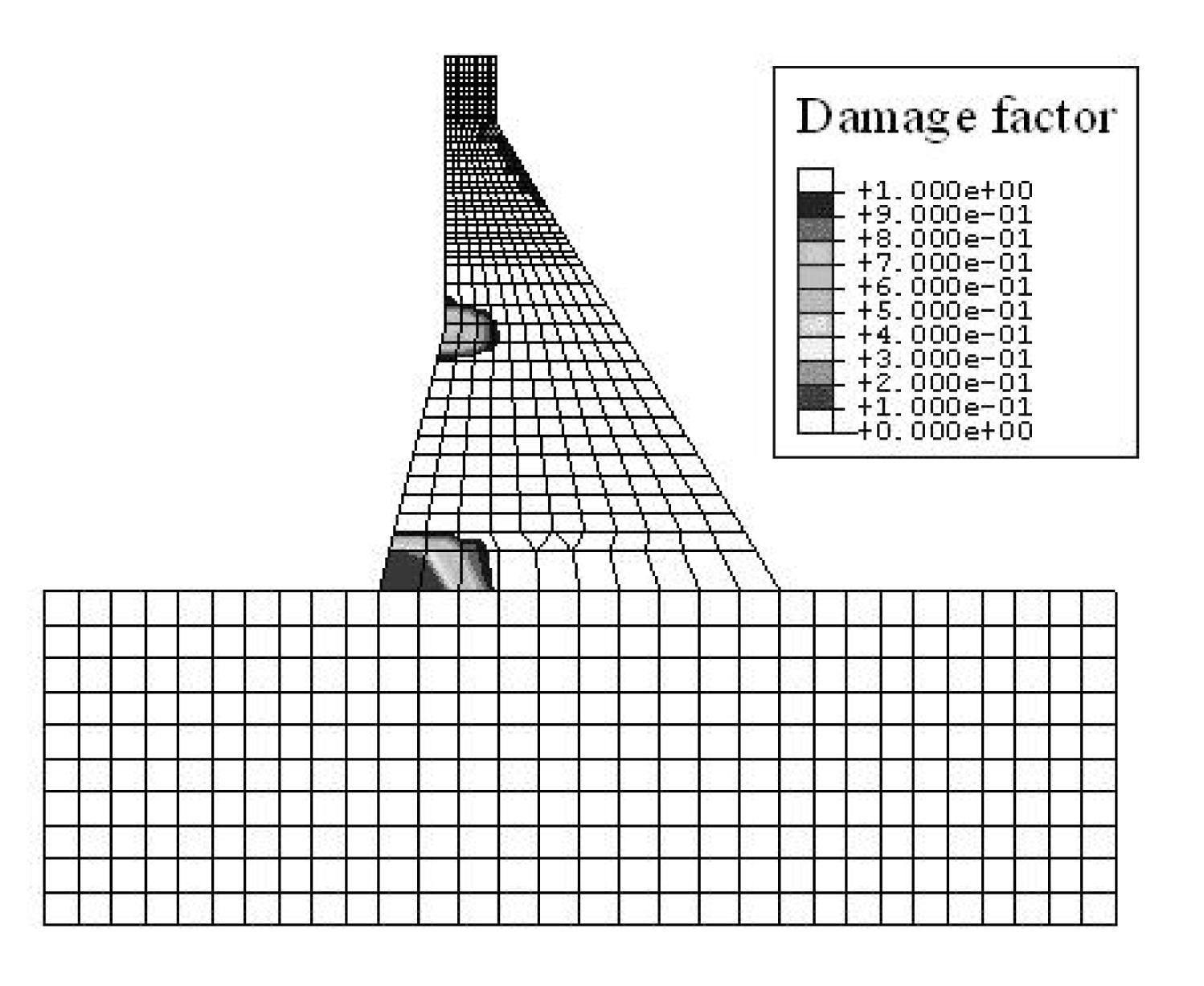Fig.8
Damage factor contour map of the slice of dam using the damage model proposed in software Abaqus/CAE

### 3.3.  Model applied in arch dam

The Baihetan arch dam which is 269 m in height and 715.3 m in arc length of the dam crest is analyzed. The thicknesses at the crest and base (maximum) of the dam are 14 and 60 m, respectively. The total number of contraction joints in the dam is 11. The normal depth of reservoir water is 260 m. The dam is located in an extremely strong earthquake region with the design peak ground acceleration (PGA), PGA=0.325g. Safety evaluation of the dam subjected to the design earthquake is a crucial factor for the project. The types of rock foundation are adopted according to rock foundation using massless foundation. The hydrodynamic pressure effects are considered using additional mass elements according to the Westergaard expression proposed by Westergaard (1933) for incompressible reservoir fluid. The normalized acceleration time course is shown in Fig. 9. The model of the Baihetan arch dam can be found in Fig. 10. The density of the dam ρ=2400 kg/m3, and Poisson’s ratio v=0.167. The damping ratio of dam ζ=0.05. The density of rock foundation ρ=2800 kg/m3, and Poisson’s ratio v=0.24. The elastic moduli of rock foundation and concrete are 20 and 25 GPa, respectively. The compressive strength fc=12.8 MPa, Poisson’s ratio λ=0.25. The parameters a, b, c, and d are 2.0006, 0.9814, 9.1312, and 0.22120, respectively, and w=1×10−6.Fig.9
Normalized acceleration time course of acceleration along the river (a), across the river (b) and in vertical direction (c) (unit: m/s2)

From the analyses of Figs. 1114, it can be observed that the maximum principal tensile stress is concentrated in the arch abutment and the top of the upstream face. The maximum principal compressive stress is concentrated in the arch abutment of the downstream face. The damage is concentrated in the arch abutment of the upstream face and the top of the downstream face. The distributions of damage calculated by the damage model in software Abaqus/CAE and the proposed damage model are similar. The maximum value of damage calculated by the damage model proposed in this paper is 0.453. The maximum value of damage calculated by the damage model proposed in software Abaqus/CAE is 0.461. It shows that the maximum value of damage of the arch dam calculated by the proposed damage model is approximately 1.74% smaller than that calculated by the damage model in software Abaqus/CAE. From the above analysis, it can be obtained that this coupled damage constitutive models can be applied in concrete arch dam.Fig.11
Principal tensile stress of upstream face (a) and downstream surface (b) using massless foundation (unit: MPa)Fig.12
Principal compressive stress of upstream face (a) and downstream surface (b) using massless foundation (unit: MPa)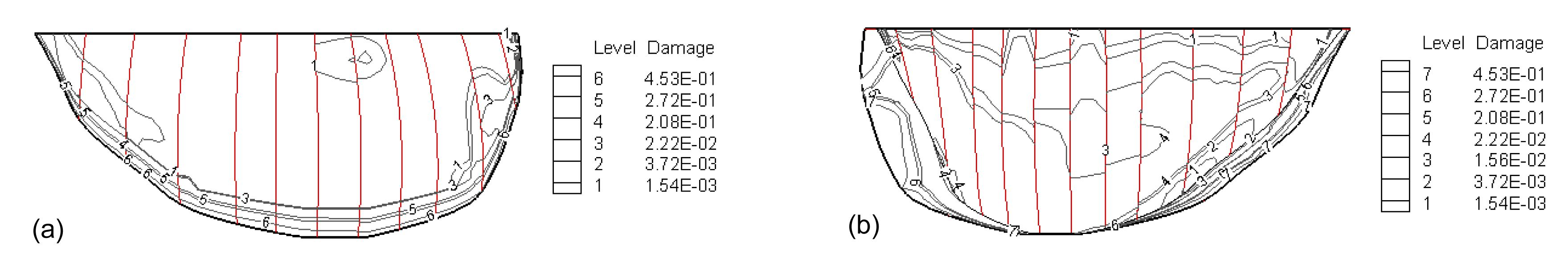Fig.13
Damage of upstream face (a) and downstream surface (b) using massless foundation proposed in this study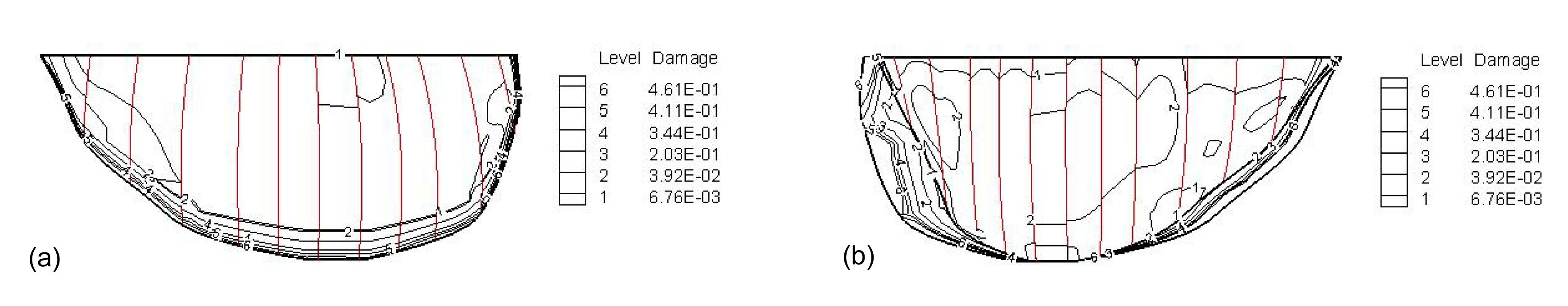Fig.14
Damage of upstream face (a) and downstream surface (b) using massless foundation using the damage model proposed in software Abaqus/CAE

## 4.  Conclusions

In order to reduce the limitation and lacuna of the traditional damage constitutive models for concrete, a new elasto-plasticity damage constitutive model for concrete is proposed based on the energy dissipation principle using the Hsieh-Ting-Chen four-parameter yield function that can reflect different strength characteristics of concrete in tension and compression. Moreover, numerical test for concrete stress-strain relation under uniaxial tension and compression is given. Compared with other damage constitutive models, the proposed model contains only one unknown parameter and the other parameters can be found in Hsieh-Ting-Chen four-parameter yield function. The same damage evolution law, which is used for tension and compression, is good for determining stress-strain constitutive and damage characteristics in complex stress state. This coupled elasto-plasticity damage constitutive model can also be applied in concrete gravity dam and arch dam.

* Project supported by the National Natural Science Foundation of China (Nos. 51109029, 51178081, 51138001 and 51009020), the China Postdoctoral Science Foundation (No. 20110491535), and the State Key Development Program for Basic Research of China (No. 2013CB035905)

## ReferencesAhmadi, M.T., Izadinia, M., Bachmann, H., 2001. A discrete crack joint model for nonlinear dynamic analysis of concrete arch dam. Computers & Structures, 79(4):403-420.Aifantis, E.C., 1999. Strain gradient interpretation of size effects. International Journal of Fracture, 95(1-4):299-314.Bazant, Z.P., Xiang, Y., Prat, P.C., 2012. Microplane model for concrete. I: stress-strain boundaries and finite strain. Journal of Engineering Mechanics, 122(3):245-254.Brencich, A., Gambarotta, L., 2001. Isotropic damage model with different tensile-compressive response for brittle materials. International Journal of Solids and Structures, 38(34-35):5865-5892.Calayir, Y., Karaton, M., 2005. A continuum damage concrete model for earthquake analysis of concrete gravity dam-reservoir systems. Soil Dynamics and Earthquake Engineering, 25(11):857-869.Chen, X.Z., Zhu, H.P., Chen, C.Y., 2005. Structural damage identification using test static data based on grey system theory. Journal of Zhejiang University-SCIENCE, 6A(8):790-796.Comi, C., Perego, U., 2001. Fracture energy based bi- dissipative damage model for concrete. International Journal of Solids and Structures, 38(36-37):6427-6454.Contrafatto, L., Cuomo, M., 2006. A framework of elastic-plastic damaging model for concrete under multiaxial stress states. International Journal of Plasticity, 22(12):2272-2370.Ding, F.X., Yu, Z.W., 2007. Strength criterion for plain concrete under multiaxial stress states based on damage Poisson’s ratio. Acta Mechanica Solida Sinica, 28(1):13-19.Dragon, A., Halm, D., Dsoyer, T., 2000. Anisotropic damage in quasi-brittle solids: modelling, computational issues and applications. Computer Methods in Applied Mechanics and Engineering, 183(3-4):331-352.Krajcinovic, D., 1983. Constitutive equation for damaging platerials. Journal of Applied Mechanics, 50(2):355-360.Kratzig, W.B., Polling, R., 2004. An elasto-plastic damage model for reinforced concrete with minimum number of material parameters. Computers and Structures, 82(15-16):1201-1215.Kuna-Ciskal, H., Skrzypek, J.J., 2004. CDM based modelling of damage and fracture mechanisms in concrete under tension and compression. Engineering Fracture Mechanics, 71(4-6):681-698.Lin, G., Hu, Z.Q., 2005. Earthquake safety assessment of concrete arch and gravity dams. Earthquake Engineering and Engineering Vibration, 4(2):251-264.Loland, K.E., 1980. Continuous damage model for load response estimation of concrete. Cement and Concrete Research, 10(1):392-492.Marfia, S., Rinaldi, Z., Sacco, E., 2004. Softening behavior of reinforced concrete beams under cyclic loading. International Journal of Solids and Structures, 41(11-12):3293-3316.Mazars, J., Pijaudier-Cabot, G., 1989. Continuum damage theory-application to concrete. Engineering Machine, 115(2):345-365.Mirzabozorg, H., Ghaemian, M., 2005. Non-linear behavior of mass concrete in three-dimensional problems using a smeared crack approach. Earthquake Engineering & Structural Dynamics, 34(3):247-269.Ortiz, M., 1985. A constitutive theory for the inelastic behavior of concrete. Mechanics of Materials, 4(1):67-93.Pan, J.W., Zhang, C.H., Wang, J.T., Xu, Y.J., 2009. Seismic damage-cracking analysis of arch dams using different earthquake input mechanisms. Science in China Series E: Technological Sciences, 52(2):518-529.Peng, X.H., Yang, C.H., Wan, L., 2004. Simplified continuum damage mechanics model for concrete. Chinese Journal of Rock Mechanics and Engineering, (in Chinese),23(19):3232-3239.Tao, X.Y., Phillips, D.V., 2005. A simplified isotropic damage model for concrete under bi-axial stress states. Cement and Concrete Composites, 27(6):716-726.Wang, G.L., Pekau, O.A., Zhang, C.H., Wang, S.M., 2000. Seismic fracture analysis of concrete gravity dams based on nonlinear fracture mechanics. Engineering Fracture Mechanics, 65(1):67-87.Zhang, D.S., 1982. Test research on complete stress-strain curve of concrete. Journal of Hunan University, (in Chinese),9(4):58-72.